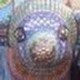# The Unapologetic Mathematician

## Decomposing the Left Regular Representation

Let’s take the left regular representation of a finite group$G$ on its group algebra$\mathbb{C}[G]$ and decompose it into irreducible representations.

Our first step is to compute the character of$\mathbb{C}[G]$ as a left$G$-module. The nice thing here is that it’s a permutation representation, and that means we have a shortcut to calculating its character:$\chi(g)$ is the number of fixed point of the action of$g$ on the standard basis of$\mathbb{C}[G]$. That is, it counts the number of$h\in G$ with$gh=h$. But this can only happen if$g$ is the group identity, and in that case every element is a fixed point. Thus we conclude\displaystyle\begin{aligned}\chi(e)&=\lvert G\rvert\\\chi(g)&=0\qquad g\neq e\end{aligned}

Now let$V$ be any irreducible representation of$G$, with character$\chi_V$. We know that the multiplicity of$V$ in$\mathbb{C}[G]$ is given by the inner product$\langle\chi_V,\chi\rangle$. This, we can calculate:\displaystyle\begin{aligned}\langle \chi_V,\chi\rangle&=\frac{1}{\lvert G\rvert}\sum\limits_{g\in G}\overline{\chi_V(g)}\chi(g)\\&=\frac{1}{\lvert G\rvert}\overline{\chi_V(e)}\lvert G\rvert\\&=\dim(V)\end{aligned}

where in the last line we use the fact that evaluating the character of any representation at the identity element gives the degree of that representation.

So, what does this tell us? Every irreducible representation$V$ shows up in$\mathbb{C}[G]$ with a multiplicity equal to its degree. In particular, it must show up at least once. That is, the left regular representation contains all the irreducible representations.

Thus if$V^{(i)}$ are the$k$ irreducible representations of$G$, we have a decomposition.$\displaystyle\mathbb{C}[G]\cong\bigoplus\limits_{i=1}^k\dim\left(V^{(i)}\right)V^{(i)}$

Taking dimensions on either side, we find$\displaystyle\lvert G\rvert=\sum\limits_{i=1}^k\dim\left(V^{(i)}\right)\dim\left(V^{(i)}\right)=\sum\limits_{i=1}^k\dim\left(V^{(i)}\right)^2$

We can check this in the case of$S_3$ and$S_4$, since we have complete character tables for both of them:\displaystyle\begin{aligned}3!=6&=1^2+1^2+2^2\\4!=24&=1^2+1^2+2^2+3^2+3^2\end{aligned}

November 17, 2010 -

1. Ah! Neat!Comment by Joe English | November 17, 2010 | Reply

2. […] Since the left regular representation is such an interesting one — in particular since it contains all the irreducible representations — we want to understand its endomorphisms. That is, we […]

Pingback by The Endomorphism Algebra of the Left Regular Representation « The Unapologetic Mathematician | November 18, 2010 | Reply

3. […] is equal to the number of irreducible representations that show up in . In particular, since we know that every irreducible representation shows up in the left regular representation , the number of […]

Pingback by The Character Table is Square « The Unapologetic Mathematician | November 19, 2010 | Reply

4. […] that , where is the number of standard tableaux of shape . Further, since we know from our decomposition of the left regular representation that each irreducible representation of shows up in with a multiplicity equal to its dimension, […]

Pingback by Standard Polytabloids Span Specht Modules « The Unapologetic Mathematician | February 18, 2011 | Reply

5. […] But , which means each irreducible -module shows up here with a multiplicity equal to its dimension. That is, is always the left regular representation. […]

Pingback by More Kostka Numbers « The Unapologetic Mathematician | February 18, 2011 | Reply